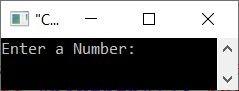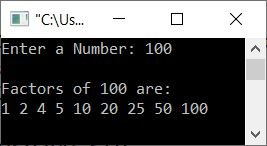# C++ Program to Find and Print Factors of a Number

This article provides some programs in C++ that find factors of a given number. For example, if the user enters 10 as input, then the program prints its factors, which will be 1, 2, 5, and 10. These are the lists of programs covered in this article:

## Find the factors of a number using the while loop

The question is, "Write a program in C++ that receives a number from the user as input and prints all its factors as output." The answer to this question is the program given below:

```#include<iostream>

using namespace std;
int main()
{
int num, i=1;
cout<<"Enter a Number: ";
cin>>num;
cout<<"\nFactors of "<<num<<" are:\n";
while(i<=num)
{
if(num%i==0)
cout<<i<<" ";
i++;
}
cout<<endl;
return 0;
}```

The snapshot given below shows the initial output produced by the above C++ program on finding factors of a given number:Now supply the input, say 100, and press the ENTER key to find and print all its factors as shown in the snapshot given below:When the user enters a number, say 100, it gets initialized to num. Therefore num=100. Now execute the following block of code from the preceding program:

```   while(i<=num)
{
if(num%i==0)
cout<<i<<" ";
i++;
}```

goes as follows (with i set to 1 and num set to 100):

• The condition i<=num or 1<=100 evaluates to true.
• Therefore, program flow goes inside the loop.
• The condition num%i==0 or 100%1==0 or 0==0 evaluates to true.
• Therefore, program flow goes inside the body of if, and the cout statement gets executed, which prints the value of i and a blank space.
• So 1 gets printed.
• The value of i is now increased. So i=2
• Again, the condition of the while loop gets evaluated.
• In other words, the condition i<=num or 2<=100 evaluates to true once more.
• Therefore, program flow again goes inside the loop.
• This process continues until the condition evaluated as false.
• In this way, all the factors of a given number get printed one by one.

## Find factors of a number using the for loop

Let's create the same program as the previous program, but using a for loop this time.

```#include<iostream>

using namespace std;
int main()
{
int num, i;
cout<<"Enter a Number: ";
cin>>num;
cout<<"\nFactors of "<<num<<" are:\n";
for(i=1; i<=num; i++)
{
if(num%i==0)
cout<<i<<" ";
}
cout<<endl;
return 0;
}```

## Find factors of a number using a function

This program is created using a user-defined function named fact(). This function takes a given number as an argument and finds and prints the factors of the passed argument.

```#include<iostream>

using namespace std;
void fact(int);
int main()
{
int num;
cout<<"Enter a Number: ";
cin>>num;
cout<<"\nFactors of "<<num<<" are:\n";
fact(num);
cout<<endl;
return 0;
}
void fact(int n)
{
int i=1;
while(i<=n)
{
if(n%i==0)
cout<<i<<" ";
i++;
}
}```

## Find factors of a number using class and object

This is the last program of this article, created using classes, an object-oriented feature of C++.

```#include<iostream>
using namespace std;

class CodesCracker
{
private:
int i;
public:
void fact(int n)
{
for(i=1; i<=n; i++)
{
if(n%i==0)
cout<<i<<" ";
}
}
};

int main()
{
CodesCracker obj;
int num;
cout<<"Enter a Number: ";
cin>>num;
cout<<"\nFactors of "<<num<<" are:\n";
obj.fact(num);
cout<<endl;
return 0;
}```

C++ Quiz

« Previous Program Next Program »